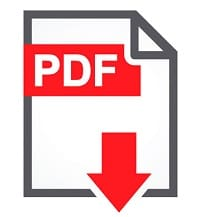# SharePoint 2013 Arranging Choice Field CheckBoxes on SharePoint Forms using jQuery

In this article, we will explore how to Arranging Choice Field’s CheckBoxes on SharePoint 2013 or SharePoint online Forms. This is always been a problem if a choice field in SharePoint is set to Check Box and the choice field values are in a large number, then the field values are arranged vertically.

The vertical arrangement makes the form look longer and hence the scrollbar gets added to the form. Viewing the form the user’s point of view the form looks quiet long.

So, I have made an effort to arrange the checkboxes (choice values) horizontally. I am sharing this with a motive to save your time and make the form appear much better.

Also read some SharePoint 2013 jquery tutorials:
Different operations Using JQuery like hide show disable and set specific value to html control

Code Implementation:
Actual look of the choice field is as shown below

In the below code example, I have put everything into a function where I first find out the total number of choice values by picking the selector and crawling through the hierarchy of HTML tags to get “.ms-RadioText”.

And then, get the total by using length.

var len = \$(“#trainTopic table tbody tr td .ms-RadioText”).length;

Then, decide on how many values you need in one row. The loops used in code are as shown below.

Outer Loop: for (i = 0; i < len; i = i + 4)
Inner Loop: for (j = i + 1; j <= i + 3; j++)

The numbers 4 and 3 in outer and inner loops respectively state that 4 columns will be created or we can say 4 choice values will be put in each row created.

You, then, loop through the full length of choice values and the iterations of this loop will take a jump of 4 counts as the number of columns I need is just 4.

And in every iteration of the outer loop, I find the row in which the 4th + 1 choice value resides in by picking the choice value in the list and find the <tr></tr> in which it stands.

The inner loop arranges the next 3 choice values i.e. the <td></td> in which it resides in the same row as the first choice value of the next row.

And, here we go.

function ArrangeCheckBoxes() {

var len = \$(“#choiceField table tbody tr td .ms-RadioText”).length;

temp = len – 1;

count = 0;

var startingElement;

for (i = 0; i < len; i = i + 4) {

if (count == temp) break;

startingElement = \$(“#choiceField table tbody tr td .ms-RadioText:eq(” + i + “)”).closest(‘tr’)

count++;

for (j = i + 1; j <= i + 3; j++) {

if (count == temp) break;

\$(“#choiceField table tbody tr td .ms-RadioText:eq(” + (j) + “)”).closest(‘td’).appendTo(startingElement);

count++;

}

}

}

Modified look of the choice field is shown below:

I hope this was helpful and saves some of your valuable time.•KK says:
•Jeseena says: